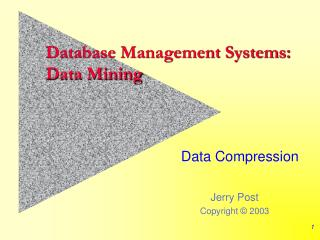DownloadDownload PresentationDatabase Management Systems: Data Mining

# Database Management Systems: Data Mining

Télécharger la présentation## Database Management Systems: Data Mining

- - - - - - - - - - - - - - - - - - - - - - - - - - - E N D - - - - - - - - - - - - - - - - - - - - - - - - - - -
##### Presentation Transcript

1. Database Management Systems:Data Mining Data Compression

2. The Need for Data Compression • Noisy data • Need to combine data to smooth it (bins) • Too many values in a dimension • Need to combine data to get smaller number of sets • Hierarchies • Rollup data into natural hierarchies • Create additional hierarchies • Data compression • Large text, images, time series (wavelet compression)

3. Bins Data: 3, 9, 17, 27, 32, 32, 37, 48, 55 • Numerical data • Create bins • Equal-depth partitions • Equal-width partitions • Bin mean/smoothing • Choose number of bins? Bin 1: 3, 9, 17 Bin 2: 27, 32, 32 Bin 3: 37, 48, 55 Bin 1: 3 – 20.3 3, 9, 17 Bin 2: 20.3 – 37.6 27, 32, 32, 37 Bin 3: 37.6 – 55 48, 55 Bin 1: 9.6, 9.6, 9.6 Bin 2: 30.3, 30.3, 30.3 Bin 3: 46.6, 46.6, 46.6

4. What is Cluster Analysis? • The process of placing items into groups, where items within a group are similar to each other, and dissimilar to items in other groups. • Similar to Classification Analysis, but in classification, the group characteristics are known in advance (e.g., borrowers who successfully repaid loans).

5. Clustering • Find groups statistically • Eliminate outliers

6. Standardization • If there are multiple attributes with continuous variables, you should standardize them. • Z-Scores are a common approach, but using a standard squared-deviation places too much weight on outliers.

7. Distance Measure • A simple distance measure is the l1 norm: • D(i,j) = |xi1-xj1| + |xi2-xj2| + … + |xin-xjn| • A more common measure is the Euclidean or l2 norm: • D(i,j) = sqrt((xi1-xj1)2 + (xi1-xj1)2 + … + (xi1-xj1)2) 3 5 x2 x1

8. General Distance Measure • In general form:

9. Hierarchies Dates Year – Quarter – Month – Day Year – Quarter – Week – Day Business hierarchies Division/Product Function: Marketing, HRM, Production, … Region Region hierarchies World – Continent – Nation – State – City

10. Clustering: Principal Components • Find the primary factors that define the data (vectors Y1, Y2) • Statistical packages can compute them quickly. • Map raw data (x1, x2 points) into the two vectors.. • Use the vectors instead of the raw data. X2 Y1 Y2 X1

11. Factor Analysis: Latent Variables • Some data is not observable, but can be measured through indicator variables. Classic example: human intelligence which can be evaluated through a variety of tests IQ Test Intelligence SAT ACT

12. Exploratory Factor Analysis • Survey (marketing) • Many items (questions) • Are they related? • Can the data be described by a small number of factors? Q1 Factor 1 Q2 Q3 Q4 Factor 2 Q5 Q6

13. Regression to Reduce Values • Estimate regression coefficients • If good fit, use the coefficients to pick categories

14. Wavelet Compression • Find patterns (wavelets) in the data • Standard compression methods (GIF) • Reduces data to a small number of wavelet patterns • Particularly useful for complex data

15. Clustering Technologies • K-means • User specifies number of clusters (k) • System minimizes intracluster distance (usually L2: sum of squared errors). • K-medoids (selects representative center point) • Hierarchical (BIRCH) • Non-spherical (CURE) • Density-based (DBSCAN, DENCLUE [best of group]) • Grid-based (STING, CLIQUE) Data Mining, Jiawei Han and Micheline Kamber, 2001; chapter 8

16. Classification • Classify an outcome based on attributes. Similar to prediction and attribute valuation, but generally splits attributes into groups. • Example: Decision Tree • Will customers buy a new item? Age <=25 >50 26…50 Yes Student Income No Yes Low High No Yes No Yes

17. Decision Tree • Modeler specifies • The dependent variable (outcome) • The attribute variables • Sample/training data • The system selects the nodes and the criteria to best fit the model. Possibly using the gain in information criteria. • Results are useful in data mining/OLAP/cube browser. The tree nodes become important sides of the cube, and the classification levels specify hierarchies.

18. Bayesian Classifier • Goal: predict the probability that an item belongs to a class. • Begin with the naïve assumption: with m classes, the a priori probability P(Ci) = 1/m • Bayes’ Theorem: The probability that sample X belongs in Class i is P(Ci|X). • Assume the attributes are independent, so you can multiply the probabilities. Estimate the attribute probabilities from the sample. For categorical data, sik is the number of training samples of Class Ci having the value xk for Ak and si is the number of training samples in Ci. For continuous variables, use a Gaussian (normal) distribution.

19. Neural Networks Output Cells Input weights 7 3 -2 4 Hidden Layer Some of the connections Incomplete pattern/missing inputs. Sensory Input Cells

20. Neural Networks: Back Propogation • Training sample with known results • Define the network • Number of layers (You must have one hidden layer) • Number of cells in each layer • Normalize the data between 0.0 and 1.0 • Initialize the weights (small random numbers) • Propagate forward and compute the error • Update the weights and biases working backwards w0j X0 Σ θj w1j X1 Output f wnj bias Xn Weighted sum Inputs

21. NN: Error Calculations • Errj = Oj (1-Oj)(Tj-Oj) • Tj is the true output, Oj (1-Oj ) is the derivative of the logistic function • For the hidden layer: • (l) is the learning rate